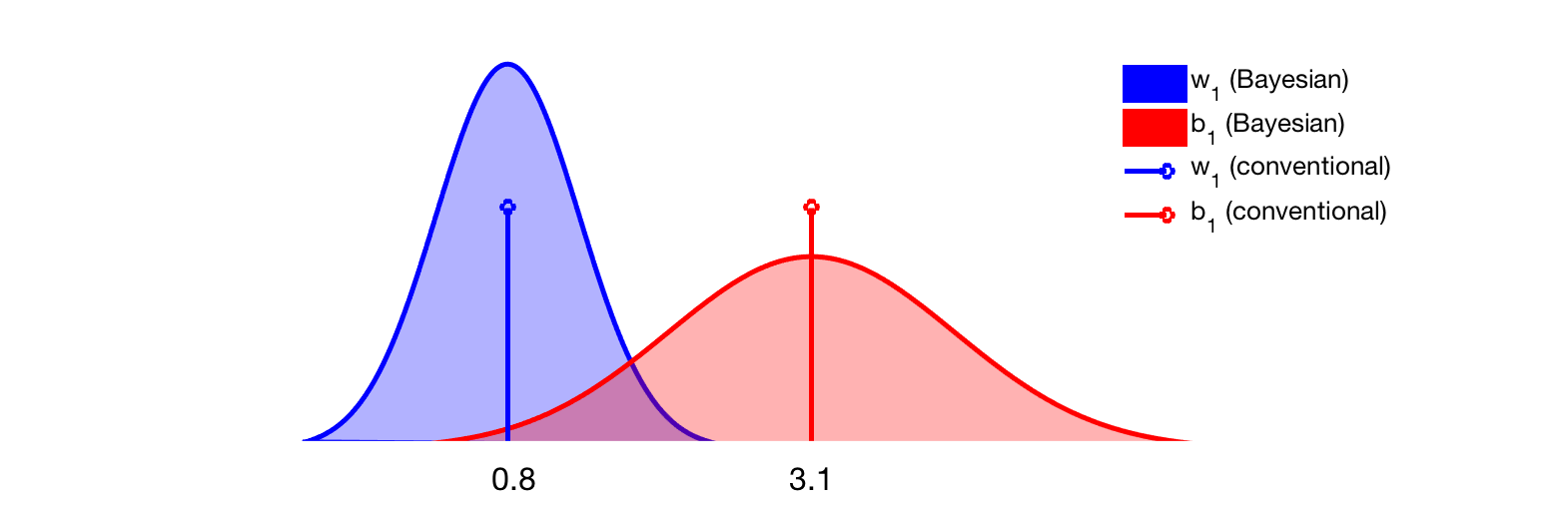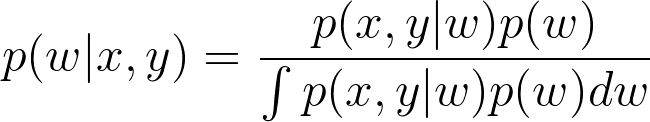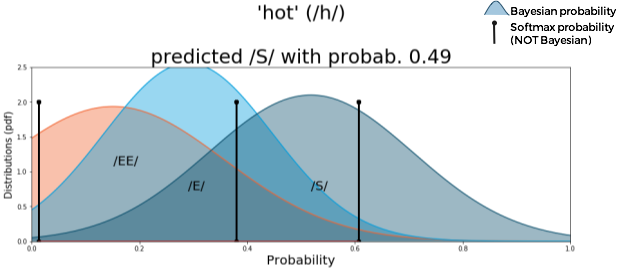# Deep Bayesian Neural Networks.

ways and whys.

Conventional neural networks aren’t well designed to model the uncertainty associated with the predictions they make. For that, one way is to go full Bayesian.

Here’s my take on three approaches.

1. Approximating the integral with MCMC
2. Using black-box variational inference (with `edward`)
3. Using MC dropout

But first. What are we trying to do?
Any deep network has parameters, often in the form of weights (`w_1`, `w_2`, …) and biases (`b_1`,`b_2`, …). The conventional (non-Bayesian) way is to learn only the optimal values via maximum likelihood estimation. These values are scalars, like `w_1 = 0.8` or `b_1 = 3.1`.
On the other hand, a Bayesian approach is interested in the distributions associated with each parameter. For instance, the two parameters above might be described by these two Gaussian curves after a trained Bayesian network has converged.Representation of two parameters (w_1 and b_1)obtained after training a Bayesian net (which will lead to parameter distributions shown by the Gaussian curves) or a conventional net (where parameters are indicated by single lines). Note, a deep neural networks has thousands if not millions of these parameters!

Having a distribution instead of a single value is a powerful thing. For one, it becomes possible to sample from a distribution many many times and see how this affects the predictions of the model. If it gives consistent predictions, sampling after sampling, then the net is said to be “confident” about its predictions.

The pain point
Estimating those distributions, of course, is the hard part. These are generally referred to as posterior densities, and are estimated using the Bayes rule.Bayes rule. x and y are input and output. The integral in the denominator requires marginalization over all possible values that the parameters “w” can assume in the model — this become quickly too hard to compute.

The problem is the denominator — also known as model evidence. It requires integrating over all possible values of the parameters (i.e., all weights and biases space), and it is often not doable in practice.

Instead, pseudo-numerical approaches can be chosen where the solution to those integrals is approximated: option 1, 2 and 3 mentioned before.

1. Approximating integrals with MCMC.

Since computing exact integrals for the Bayes rule is hard, MCMC (Markov Chain Monte Carlo) is used to approximate these. The idea behind MCMC is really handsome and I’d suggest this uber-famous blogpost to get code and an insight behind the approach. Maths aside, however, this method is the slowest and the least sexy of the three options.

Pros: In theory MCMC eventually leads to great results, with approximations that resemble the real thing (posteriors).
Cons: In practice it takes a long time to converge, when it ever does.

2. Using black-box variational inference (eg., using `edward`).

Variational inference is an approach to estimate a density function by choosing a distribution we know (eg. Gaussian) and progressively changing its parameters until it looks like the one we want to compute, the posterior. Changing parameters no longer requires mad calculus; it’s an optimization process, and derivatives are usually easier to estimate than integrals. This “made-up” distribution we are optimizing is called variational distribution. There is some really elegant math that shows how choosing the optimal parameters for the variational distribution is equivalent to maximizing a lower bound, and definitely worth checking it up at some point. But if you want to know how to get it to work, and leave the theory aside for now, I have prepared a short tutorial for an 8-category classification task using `edward`.

`# Define:#  ~ K number of classes, e.g. 8#  ~ D size of input, e.g. 10 * 40#  ~ BATCH_SIZE, N_TRAIN, N_TEST, STEPS (how many training steps)`
`# Tensorflow Placeholders:w = Normal(loc=tf.zeros([D, K]), scale=tf.ones([D, K]))b = Normal(loc=tf.zeros(K),      scale=tf.ones(K))x = tf.placeholder(tf.float32, [None, D])y = Categorical(tf.matmul(x,w)+b)y_ph = tf.placeholder(tf.int32, [BATCH_SIZE]) # For the labels`

Setting up the variational distribution. We’ll choose Gaussians. As for inference technique, we pick one based on KL divergence. The actual estimation of the KL is carried out estimating the ELBO via a ‘black-box’ variational approach (if you care about what it means >> read this).

`qw = Normal(loc=tf.Variable(tf.random_normal([D, K])),        scale=tf.nn.softplus(tf.Variable(tf.random_normal([D, K]))))qb = Normal(loc=tf.Variable(tf.random_normal([K])),        scale=tf.nn.softplus(tf.Variable(tf.random_normal([K]))))inference = ed.KLqp({w: qw, b: qb}, data={y: y_ph})inference.initialize(n_iter=STEPS, n_print=100, scale={y: N_TRAIN / BATCH_SIZE})`

Model has been defined, we’re now ready to start a tf session and train.

`sess = tf.InteractiveSession()tf.global_variables_initializer().run()train_data_generator = generator(train_data, BATCH_SIZE)train_labs_generator = generator(train_labels, BATCH_SIZE)for _ in range(inference.n_iter):    X_batch = next(train_data_generator)    Y_batch = next(train_labs_generator)    info_dict = inference.update(feed_dict={x: X_batch, y_ph: Y_batch})    inference.print_progress(info_dict)`

One can now sample these distributions (`qw` for the weights, and `qb `for the biases) and look at the spread around each prediction, which indicates model uncertainty.

`prob_lst, samples, w_samples, b_samples = [], [], [], []for _ in range(1000):    w_samp = qw.sample()    b_samp = qb.sample()    w_samples.append(w_samp)    b_samples.append(b_samp)        # Probability of each class for each sample.    prob = tf.nn.softmax(tf.matmul( X_test, w_samp ) + b_samp)    prob_lst.append(prob.eval())    sample = tf.concat([tf.reshape(w_samp,[-1]),b_samp],0)    samples.append(sample.eval())`

You can play with `prob_lst` and `samples` for this. In conclusion:

Pros: it is faster than plain MCMC, and libraries like `edward` help getting your Bayesian net up and running in minutes.

Cons: it might get slow for very deep Bayesian net, and performance isn’t always guaranteed to be optimal.

3. Using MC dropout.

MC dropout is a recent theoretical finding that provides a Bayesian interpretation of the regularization technique known as “dropout”. The reasoning is a little as follows. Variational inference is a Bayesian approach to estimate posteriors using an arbitrary distribution, the “variational distribution” introduced earlier; the dropout, instead, is a form of regularization for neural networks where neurons are randomly turned on or off during training to prevent the network to depend on any specific neuron. And here is the key idea of MC dropout: dropout could be used to perform variational inference where the variational distribution is from a Bernoulli distribution (where the states are “on” and “off”). “MC” refers to the sampling of the dropout, which happens in a ‘Monte Carlo’ style.
In practice, turning a conventional network into a Bayesian one via MC dropout can be as simple as using dropout for every layer during training AS WELL AS testing; this is equivalent to sampling from a Bernoulli distribution and provides a measure of model’s certainty (consistency of predictions across sampling). It is also possible to experiment with other variational distributions.

Pros: It is easy to turn an existing deep net into a Bayesian one. it is faster than other techniques, and does not require an inference framework.

Cons: Of course, sampling at test time might be too expensive for computationally-demanding (eg real time) applications.

Again, what’s the big deal?
Despite all, many researchers are still not convinced that going Bayesian is worth the hassle. Let me take one more shot at this. I work in the autonomous vehicle sector, so the following is an example close to my heart. Suppose one is driving a brand new car with the latest and greatest autopilot feature, when an unidentified objects pops in front of the driver. In fact,let’s make this example unlikely but entirely possible by saying that the unidentified object is this odd flying-car (which is going to be for sale soon and yes, so you might share the road with it one day). A computer vision algorithm that has never been trained to overtake such thing, and has only seen planes up in the sky, might be thinking that the plane must be far away.
What could happen?
A conventional network might over-confidently misjudge the position of the flying-car, and do the wrong thing, while a Bayesian network has access to its uncertainty and would suggest to slow down.An real-case example of conventional Vs Bayesian approach in neural networks with similar architectures (from some of my previous working building phoneme classifiers). The conventional approach provides only the softmax probability with every prediction (in this case, predictions of the phoneme being /EE/, /E/ or /S/) and is represented by the vertical black lines. On the other hand, the Bayesian approach provides certainty estimates in the “spreads” around the softmax values, which are represented by the Gaussian curves. For this particular example, the models were trained on a number of phonemes but not on the phoneme being presented at test time (‘/h/’), therefore there is no correct answer the models can give. Note, however, how the Bayesian approach expresses high levels of uncertainty with this prediction, whereas the conventional approach show over-confidence about the prediction of phoneme ‘/S/’ being correct.